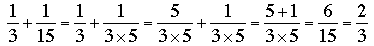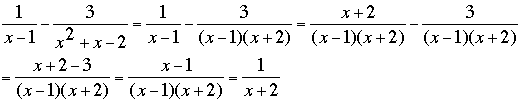SEARCH HOMEMath Central Quandaries & QueriesQuestion from Jonathon, a student: Hi, I have a question of adding polynomials that are in fraction form: The equation is: 1/(x+3) + 1/(x^2+5x+6) = Thanks, JonathanHi Jonathon,

These are not polynomials they are rational functions. A rational function is a function that can be written as a polynomial divided by a polynomial. You add rational functions just as you would add rational numbers (fractions) by first finding a common denominator. For example to add 1/3 and 1/15 I can see that 15 = 3 × 5 so 15 is a common denominator and henceNow let's try 1/(x - 1) - 1/(x2 + x - 2). Again I need a common denominator and since x2 + x - 2 = (x - 1)(x + 2) I can use x2 - x - 2 as a common denominator. ThusNow you try 1/(x+3) + 1/(x^2+5x+6) .

If you need further assistance write back.
PennyMath Central is supported by the University of Regina and The Pacific Institute for the Mathematical Sciences.